Home > INT3 > Chapter 7 > Lesson 7.2.5 > Problem7-112

7-112.
1. Solve each of the following equations for the indicated variable. Homework Help ✎

1. 5x – 3y = 12 for y

2. F =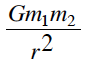for m2

3. E =mv2 for v

4. (x – 4)2 + (y – 1)2 = 10 for y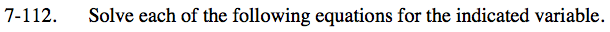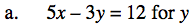Isolate y on one side of the equation.
You can leave coefficients in fraction form.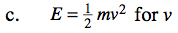Start by multiplying by 2 and dividing by m.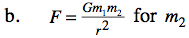$m_{2} = \frac{Fr^2}{Gm_1}$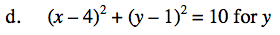$(y-1)^2=10-(x-4)^2$

$y-1=\pm\sqrt{10-(x-4)^2}$

$y=\pm\sqrt{10-(x-4)^2}+1$